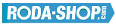# Remote ControlManufacturer Product Image Item Name Price### 602X-2Z

Miniature Metric Series Chrome Steel Metallic Shields on both sides Dimension d=2.50 Dimension D=8.00 Dimension B=4.00 Dinamic Load Rating=552 Static Load Rating=177 Weight=0.001gr. Speed limit Grease=60.0 Speed limit Oil=71.0
inside diameter = 2.50
outside diameter = 8.00
width = 4.00
€2.24### 603

Miniature Metric Series Chrome Steel Open(No Shields) Dimension d=3.00 Dimension D=9.00 Dimension B=3.00 Dinamic Load Rating=571 Static Load Rating=189 Weight=0.002gr. Speed limit Grease=56.0 Speed limit Oil=67.0
inside diameter = 3.00
outside diameter = 9.00
width = 3.00
€2.24### 603-2Z

Miniature Metric Series Chrome Steel Metallic Shields on both sides Dimension d=3.00 Dimension D=9.00 Dimension B=5.00 Dinamic Load Rating=571 Static Load Rating=189 Weight=0.002gr. Speed limit Grease=56.0 Speed limit Oil=67.0
inside diameter = 3.00
outside diameter = 9.00
width = 5.00
€2.24### 604

Miniature Metric Series Chrome Steel Open(No Shields) Dimension d=4.00 Dimension D=12.00 Dimension B=4.00 Dinamic Load Rating=957 Static Load Rating=350 Weight=0.003gr. Speed limit Grease=48.0 Speed limit Oil=56.0
inside diameter = 4.00
outside diameter = 12.00
width = 4.00
€2.24### 604-2RS

Miniature Metric Series Chrome Steel Rubber Seals on Both sides Dimension d=4.00 Dimension D=12.00 Dimension B=4.00 Dinamic Load Rating=957 Static Load Rating=350 Weight=0.003gr. Speed limit Grease=48.0 Speed limit Oil=56.0
inside diameter = 4.00
outside diameter = 12.00
width = 4.00
€2.24### 604-2Z

Miniature Metric Series Chrome Steel Metallic Shields on both sides Dimension d=4.00 Dimension D=12.00 Dimension B=4.00 Dinamic Load Rating=957 Static Load Rating=350 Weight=0.003gr. Speed limit Grease=48.0 Speed limit Oil=56.0
inside diameter = 4.00
outside diameter = 12.00
width = 4.00
€2.24### 605

Miniature Metric Series Chrome Steel Open(No Shields) Dimension d=5.00 Dimension D=14.00 Dimension B=5.00 Dinamic Load Rating=1329 Static Load Rating=507 Weight=0.005gr. Speed limit Grease=40.0 Speed limit Oil=50.0
inside diameter = 5.00
outside diameter = 14.00
width = 5.00
€2.24### 605-2RS

Miniature Metric Series Chrome Steel Rubber Seals on Both sides Dimension d=5.00 Dimension D=14.00 Dimension B=5.00 Dinamic Load Rating=1329 Static Load Rating=507 Weight=0.005gr. Speed limit Grease=40.0 Speed limit Oil=50.0
inside diameter = 5.00
outside diameter = 14.00
width = 5.00
€2.24### 605-2Z

Miniature Metric Series Chrome Steel Metallic Shields on both sides Dimension d=5.00 Dimension D=14.00 Dimension B=5.00 Dinamic Load Rating=1329 Static Load Rating=507 Weight=0.005gr. Speed limit Grease=40.0 Speed limit Oil=50.0
inside diameter = 5.00
outside diameter = 14.00
width = 5.00
€2.24### 606

Miniature Metric Series Chrome Steel Open(No Shields) Dimension d=6.00 Dimension D=17.00 Dimension B=6.00 Dinamic Load Rating=2263 Static Load Rating=846 Weight=0.007gr. Speed limit Grease=38.0 Speed limit Oil=45.0
inside diameter = 6.00
outside diameter = 17.00
width = 6.00
€2.24